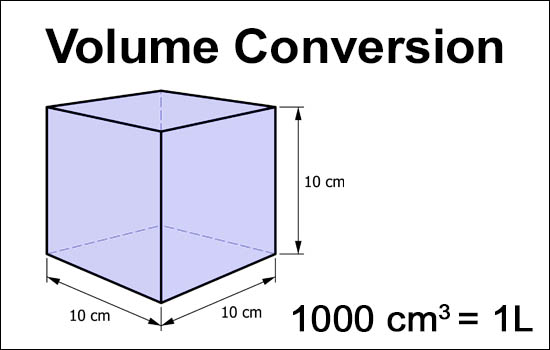# Volume Conversion Calculator

This calculator calculates and converts various volume units like liter(L), cubic meter(m³), cubic foot(ft³), gallon, quart, pint, fluid ounce, and barrel.

Output values are rounded to the sixth decimal point.From: